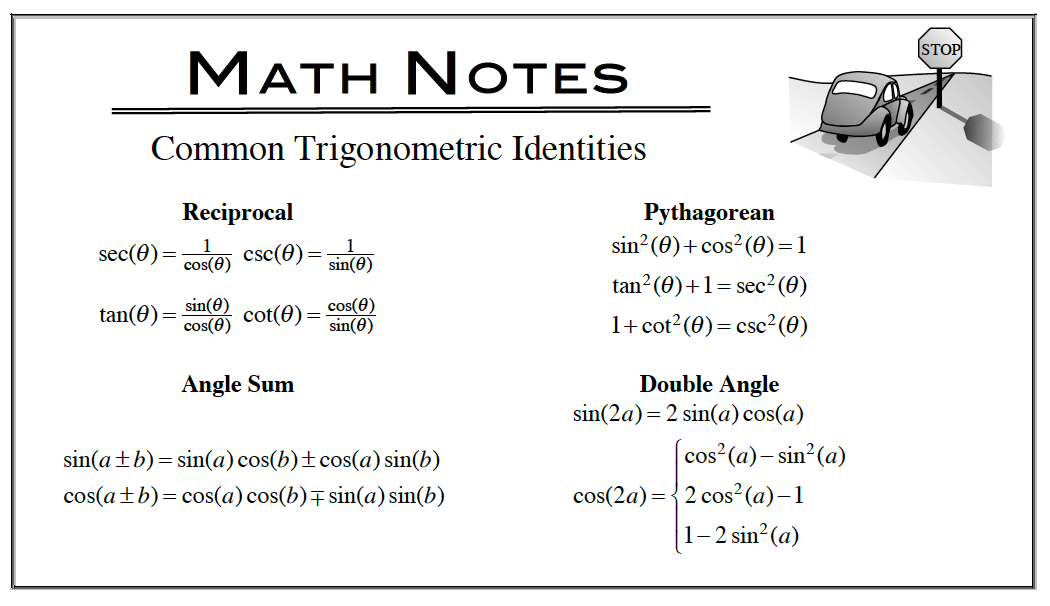### Home > APCALC > Chapter 6 > Lesson 6.3.3 > Problem6-110

6-110.

Multiple Choice: Which of the following expressions is equal to $\frac { d } { d x }\cos^2(x)$? Homework Help ✎

 I. $2 \cos(x)$ II. $–2\cos(x)\sin(x)$ III. $–\sin(2x)$
1. I only

1. II only

1. III only

1. II and III

1. none of these

There may be more than one correct answer.